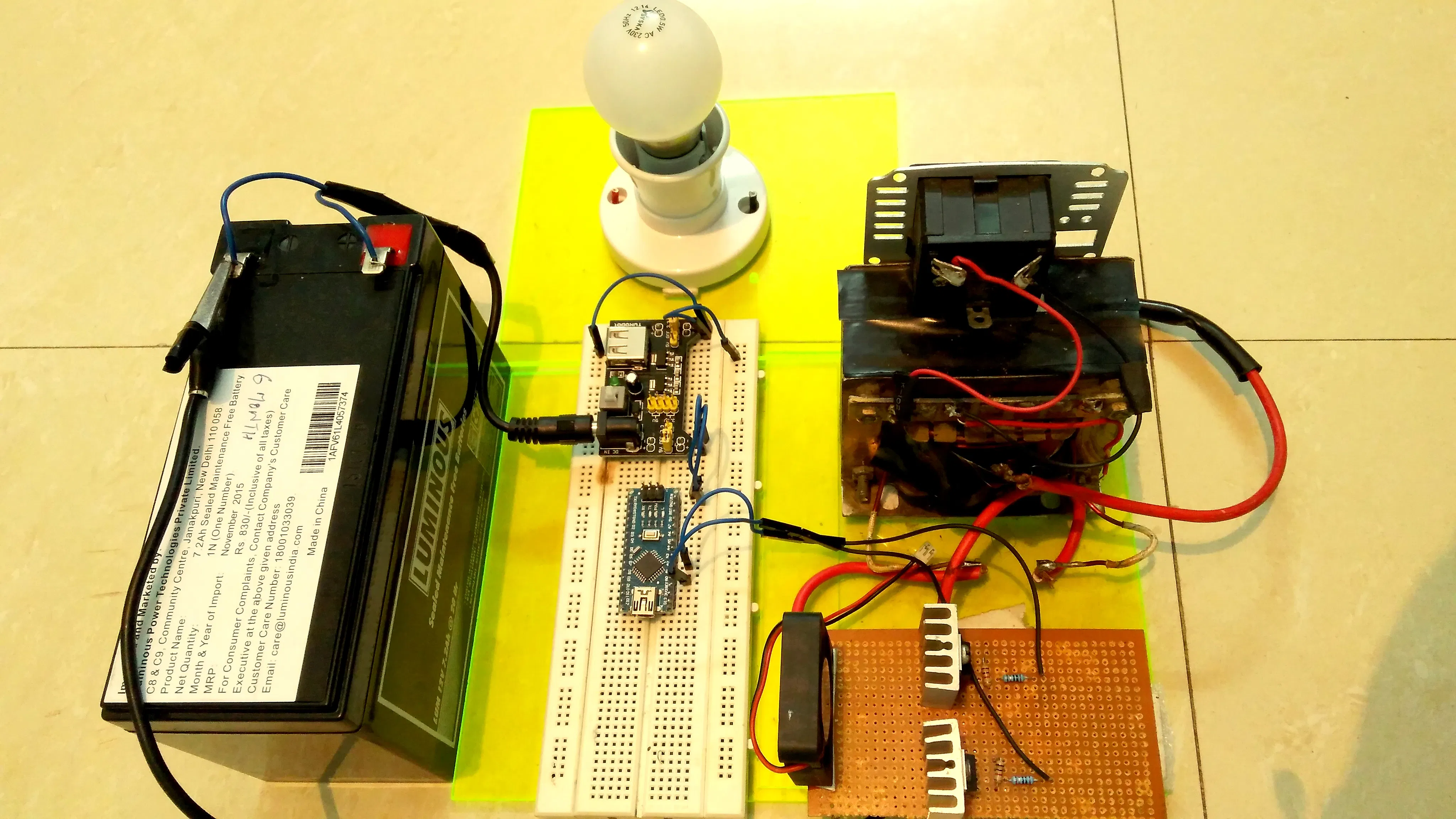Project tutorial# Make an Inverter at Home Using Arduino © GPL3+

This project is all about making a power inverter using arduino. The Inverter produces 220VAC from 12V battery.

• 6,724 views
• 12 respects

## Necessary tools and machines

 DSO
 MultimeterSoldering iron (generic)

This Project is all about making an Inverter using Arduino nano. I decided to make it because using Arduino makes it a lot easier to design the circuit as well as to diagnose it.

Basically the working of Inverter can be classified into three stages

1) Driver Stage

2) Power Stage

3) Transformer

In the driver stage Arduino is acting as an heart of the system. It is generating the modified sine waveform as well as handling all the housekeeping tasks such as battery voltage measurement etc.

In power stage the current is amplified to a required level to be fed into the transformer.

The step up transformer is used here to give an output of 220V AC.

For more details like circuit diagram and coding watch the complete video

## Code

##### InverterArduino
Its an Arduino nano Code
```#include "TimerOne.h"               // include TimerOne.h
#define low_battery_voltage 10.2               // define high battery voltage limit as 14.2
#define high_battery_voltage 14.4               // define low battery voltage limit as 10.2
int dutycycle = 0;               // Initailize duty cylce variable as integer data type
int sense_value =0;               // Initialize sense_value variable to capture the adc reading of battery voltage (range from 0 to 1023)
float battery_voltage = 0.0;               // Initialize battery_voltage variable as a float data type to convert sense_value to actual battery voltage

void setup()               // Setup function
{
pinMode (9,OUTPUT);              // set pin 9 as an output pin for pwm
pinMode (10,OUTPUT);             // set pin 10 as an output pin for pwm
Timer1.initialize(20000);             // Initailize timer1 time period as 20 milli second (50 Hz frequency)
Timer1.attachInterrupt(battery_voltage_measurement);      // battery_voltage_measurement function will be executed every 20 milli second using timer 1 overflow interrupt
TCCR1A = (TCCR1A & 0x0F) | 0xB0 ;             // set pin 10 inverted of pin 9
}

void battery_voltage_measurement()               // battery_voltage_measurement function starts
{ sense_value = (analogRead(A0));               // read battery voltage on pin A0 & capture that value in sense_value variable
// {warning - arduino pin accept only upto 5v so don't forget to map upper
//  battery volatge i.e 14.2v to 5v using voltage divider resistor network}

battery_voltage = sense_value * (14.4/1023.0);               // convert sense_value (0 to 1023) to range (0 to 14.2)
if(battery_voltage < 14.4 && battery_voltage > 10.2) // if battery voltage is under limit i.e between 10.2 and 14.2 then dutycycle will be 150
{
dutycycle = 300;
}

else if(battery_voltage < 10.2 || battery_voltage > 14.4)              // if battery voltage is below 10.2v or above 14.2v ,
{
dutycycle = 0;         //set the duty cycle to 0 and inverter will go in cutoff mode
}

}              // battery_voltage_measurement function ends

void loop()              // loop function starts
{
Timer1.pwm(9,dutycycle,20000);              // Timer1.pwm function takes argument as (pin no. , dutycycle , time period)
Timer1.pwm(10,1024-dutycycle,20000);

}               // loop function ends

```

## Schematics

Complete schematic of inverter using Arduino nano drawn in Eagle software
Inverter%20schematics.sch

June 1, 2016

#### Members who respect this project

See similar projects
you might like

#### Make your own Power Inverter using Arduino

Project showcase by Team Shailza

• 37,045 views
• 21 respects

#### Turn your RC Car to Bluetooth RC car

Project tutorial by Prajjwal Nag

• 20,674 views
• 25 respects

#### IoT Dune Buggy - Control it from Anywhere!

Project tutorial by Satyavrat Wagle

• 11,022 views
• 30 respects

#### Programmable Pocket Power Supply with OLED Display

Project tutorial by Shahariar

• 9,236 views
• 42 respects

#### DIY 3-Axis CNC VMC

Project tutorial by Amit Nandi (BigWiz)

• 22,675 views